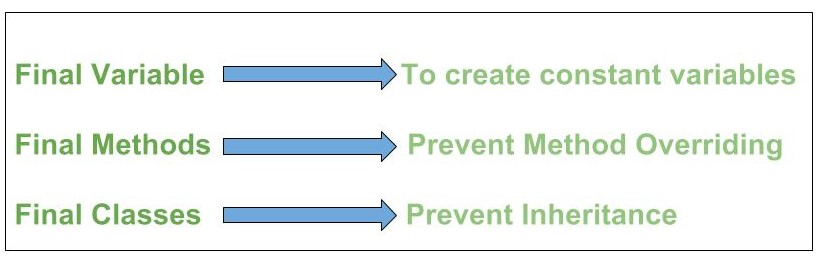# Output of Java programs | Set 24 (Final Modifier)

Difficulty level : Easy
Prerequisite : final keyword in javaPredict the output of following Java Programs:

1. What will be output of following program?

 `class` `Test  ` `{ ` `    ``final` `int` `MAXIMUM; ` `    ``final` `double` `PI; ` ` `  `public` `Test(``int` `max) ` `    ``{ ` `        ``MAXIMUM = max; ` `    ``} ` ` `  `public` `Test(``double` `pi) ` `    ``{ ` `        ``PI = pi; ` `    ``} ` ` `  `public` `static` `void` `main(String[] args) ` `    ``{ ` `        ``Test t1 = ``new` `Test(``1500``); ` `        ``Test t2 = ``new` `Test(``3.145``); ` ` `  `        ``System.out.println(``"MAXIMUM : "` `+ t1.MAXIMUM + ``" PI : "` `+ t2.PI); ` `    ``} ` `} `

a) Compilation error
b) Runtime error
c) 1500 3.145
d) 3.145 1500

`Ans. a) Compilation error`

Explanation : As we know that we can initialize a blank final variable inside constructor also, but if there are more than one constructors then it is mandatory to initialize all final variables in all of them. This is because we can create an object of class by calling any one of the constructors, but if that constructor is not initializing any one of declared final variable than there is problem.

2. What will be output of following program?

 `class` `Test  ` `{ ` `    ``final` `int` `MAXIMUM = m1(); ` ` `  `private` `int` `m1() ` `    ``{ ` `        ``System.out.println(MAXIMUM); ` `        ``return` `1500``; ` `    ``} ` ` `  `public` `static` `void` `main(String[] args) ` `    ``{ ` `        ``Test t = ``new` `Test(); ` ` `  `        ``System.out.println(t.MAXIMUM); ` `    ``} ` `} `

a) Compilation error
b) Runtime error
c) 0
1500
d) 1500
1500

`Ans. c)`

Explanation : A final variable can only be initialized once, either via an initializer or an assignment statement. Also you might think that in above program, MAXIMUM is initialized two times.This is wrong. The output is based on the fact that JVM first initialize any(final or normal) variable with it’s default-type value and then look through assignment statement(if any).

3. What will be output of following program?

 `// filename : Test1.java ` `final` `interface` `Test ` `{ ` `    ``int` `MAXIMUM = ``1500``; ` ` `  `    ``void` `m1(); ` `} ` ` `  `class` `Test1 ``implements` `Test { ` ` `  `    ``@Override` `public` `void` `m1() ` `    ``{ ` `        ``System.out.println(``"From Test1 m1 method"``); ` `    ``} ` ` `  `public` `static` `void` `main(String[] args) ` `    ``{ ` `        ``new` `Test1().m1(); ` `    ``} ` `} `

a) Compilation error
b) Runtime error
c) From Test1 m1 method

`Ans. a) Compilation error`

Explanation : Interfaces can never be declared final as they are meant to be implemented in derived classes. Please see interface and inheritance

4. What will be output of following program?

 `class` `Test { ` `public` `static` `void` `main(String[] args) ` `    ``{ ` `        ``int` `arr[] = { ``1``, ``2``, ``3` `}; ` ` `  `        ``// final with for-each statement ` `        ``for` `(``final` `int` `i : arr) ` `            ``System.out.print(i + ``" "``); ` `    ``} ` `} `

a) Compilation error
b) Runtime error
c) 1 2 3

`Ans. c) 1 2 3`

Explanation : Since the variable i goes out of scope with each iteration of the loop, it is actually getting re-declared after each iteration, allowing the same token (i.e. i) to be used to represent multiple variables.

5. What will be output of following program?

 `class` `Test { ` `public` `static` `void` `main(String[] args) ` `    ``{ ` `        ``final` `StringBuilder sb = ``new` `StringBuilder(``"Geeks"``); ` ` `  `        ``sb.append(``"ForGeeks"``); ` ` `  `        ``System.out.println(sb); ` `    ``} ` `} `

a) Compilation error
b) Runtime error
c) Geeks
d) GeeksForGeeks

`Ans. d) GeeksForGeeks`

Explanation : In case of a reference final variable(here sb), internal state of the object pointed by that reference variable can be changed. Note that this is not re-assigning. This property of final is called non-transitivity.

6. If you like GeeksforGeeks and would like to contribute, you can also write an article using write.geeksforgeeks.org or mail your article to review-team@geeksforgeeks.org. See your article appearing on the GeeksforGeeks main page and help other Geeks.